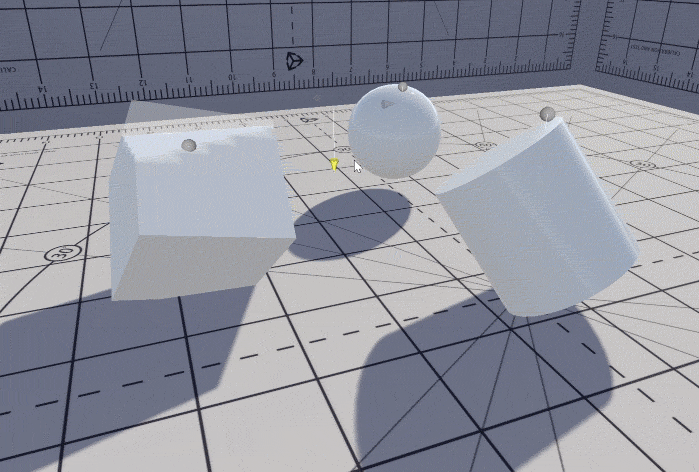# Container Water 02: Constraining the water to the bowl

As I found in the last post in this series, just pushing verts down to the water’s surface comes with an immediate issue (as seen above in the featured image).

The verts very quickly fall outside of the original volume, which breaks the effect. Now if it was a sphere, we could mitigate this by not reducing the water level below the half-way mark, but this doesn’t help if our simulation wants to displace water beyond this. So we need a solution.

In the above gif, we have a sphere, a cylinder, and a cube, but to begin with I’m going to start with a sphere. Fortunately constraining verts into a sphere is simple (Simple now after spending some time re-learning my forgotten math schooling) Pythagorean theorem – the problem breaks down into triangles.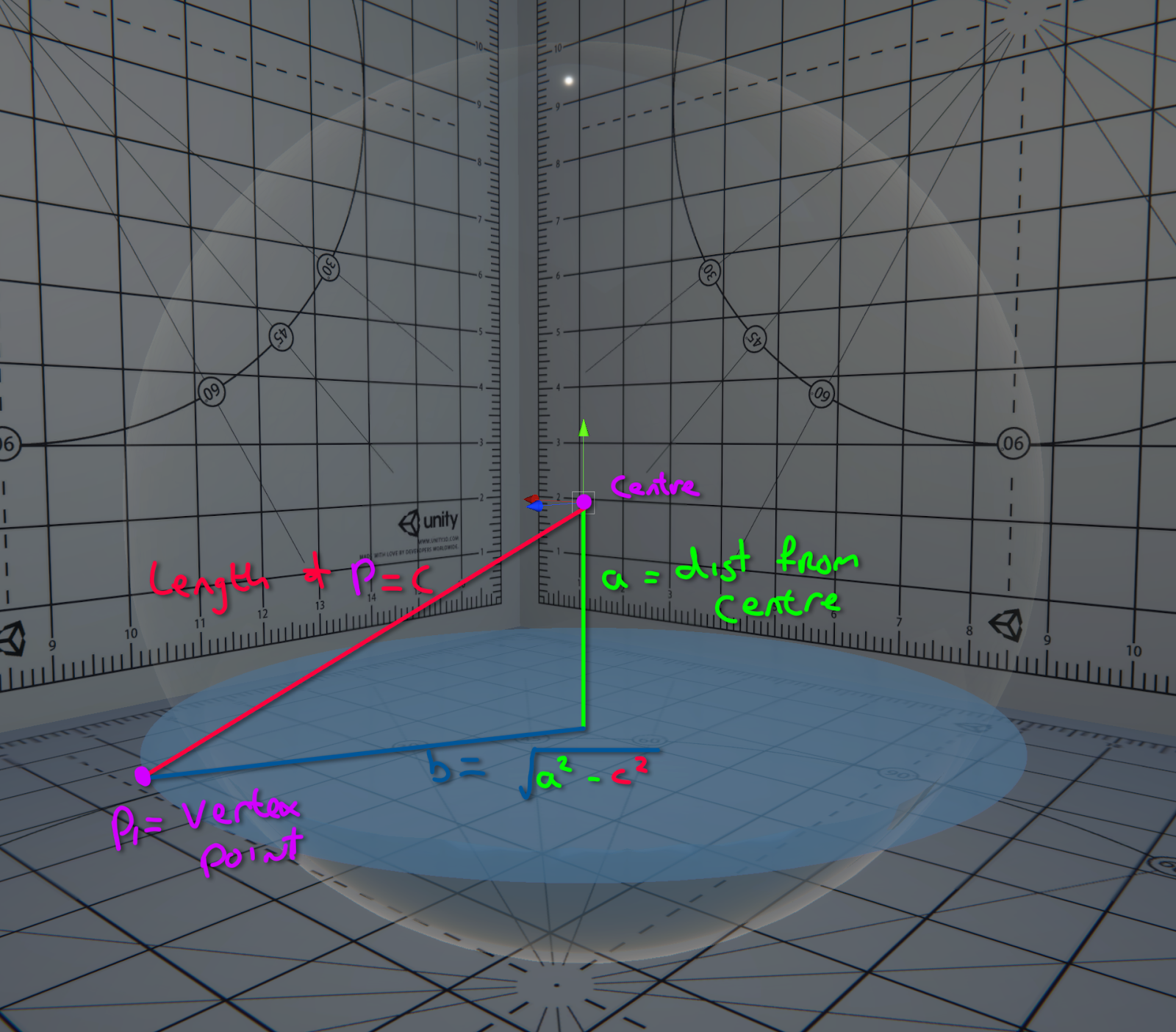First construct a triangle (abc).

Take a vector from the centre of the sphere to the vertex point you wish to constrain, this vector forms the hypotenuse ‘c’.

Side ‘a’ is the height of the water from the origin along your up axis.

Side ‘b’, the perpendicular vector to ‘a’, is the square root of ‘a’ squared minus ‘c’ squared.

This gives the length of b, which is the distance horizontally from the height of the water plane, to the vert’s actual position.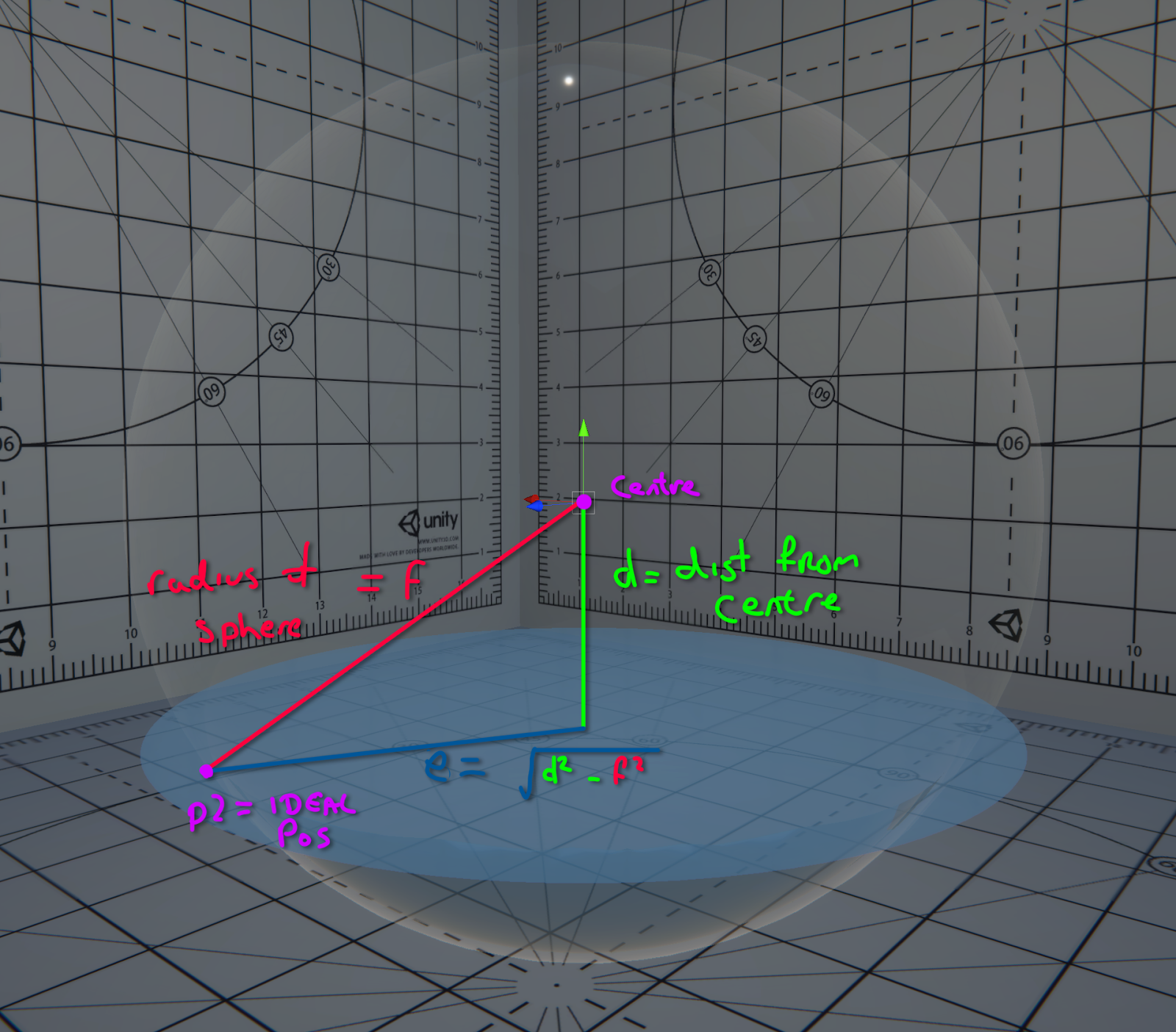Then construct another very similar triangle (def), where the hypotenuse ‘f’ is the radius of the sphere, and ‘d’ once again is the height.

Calculate ‘e’ in the same method to ‘b’ earlier, to get the furthest distance our vert can be placed before it hits the wall of our spherical container.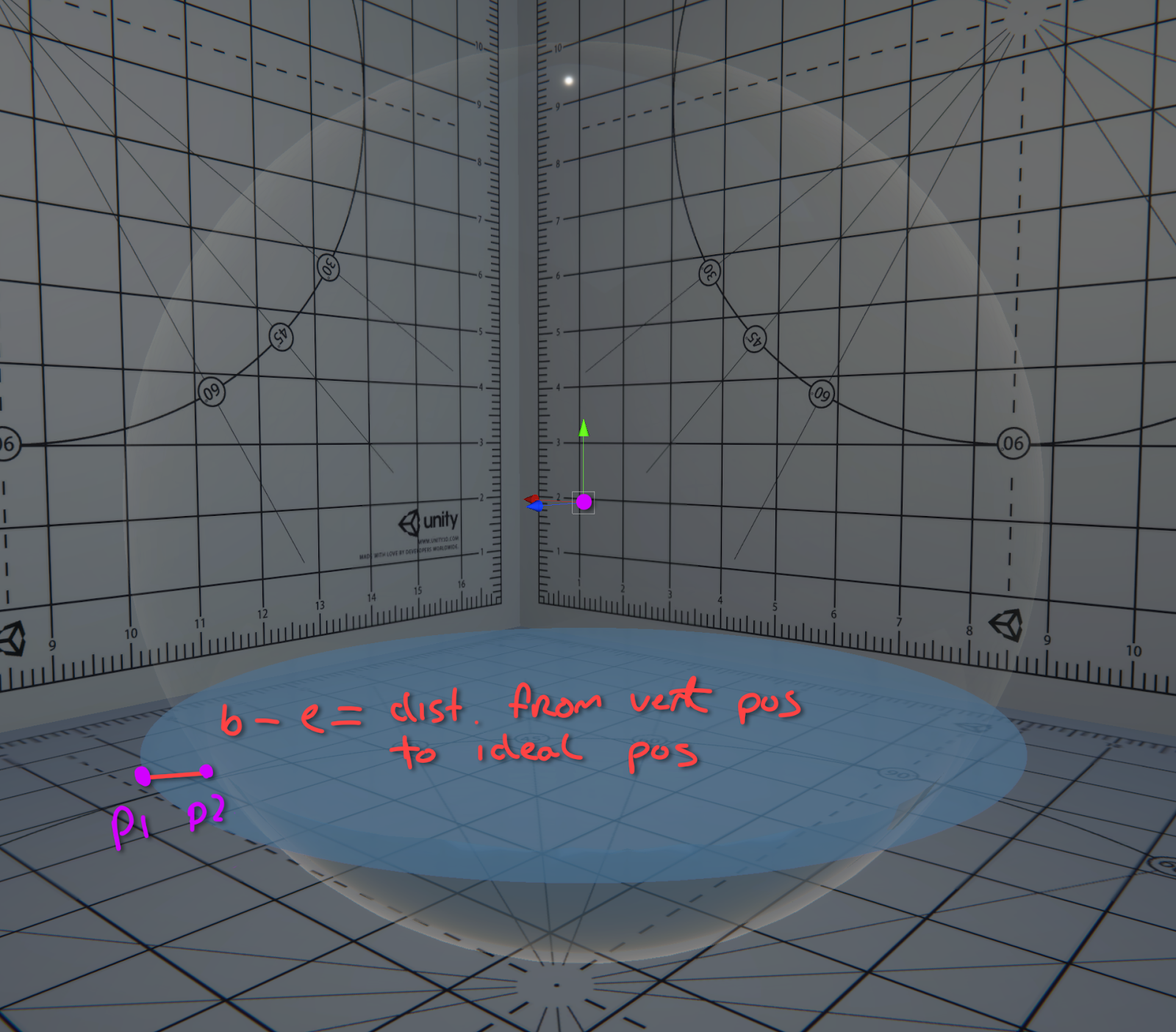Finally, translate the vertex towards the centre line by difference in length of sides ‘b’ and ‘e’.

And here’s the code, it’s structured a little differently to the explanation, but the approach is the same.

``````void ConstrainToSphere_float(float3 impactPosition,
float3 impactNormal,
float3 vertPos,
out float3 constrainedPos){

// Impact normal is central axis for sphere
// height islength of closest point along it
// https://stackoverflow.com/a/9557244 - Simplified as our
// impact normal is normalized

float height = dot(vertPos, impactNormal);
// Point along the central axis
float3 cp = impactNormal * height;

// Length of b1, where it is the vector from the center point
// of the sphere at the correct height, to the current
// vertexPosition
float3 vpcp = (vertPos - cp); // Vert to central axis
float b1 = length(vpcp);

// Length of b2, where b2 is the furthest away from the centre
// we can travel in the sphere
(height * height));

// Distance beyond the sphere our vert is
float d = b2 - b1;
// Move our vertex position towards our central axis by de
float3 outPos = vertPos + (d * normalize(vpcp));

// If the vertex is outside of the sphere's radius, move it
// towards the centre by the difference of b1 and b2
constrainedPos = length(vertPos) > sphereRadius ? outPos :
vertPos;
}

``````

Now to improve that deformation…

I’m not using Unity’s default sphere mesh any more, but an icosahedron as it has a much more uniform distribution of verts. This means we get a similarly predictable deformation all over the sphere.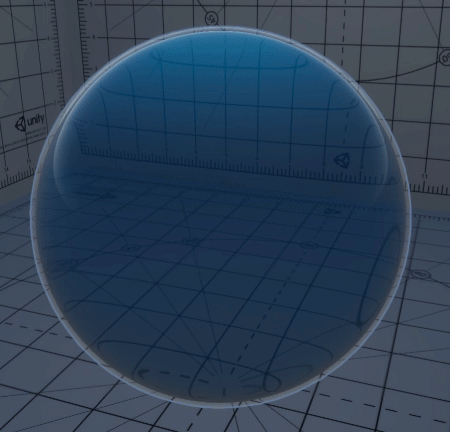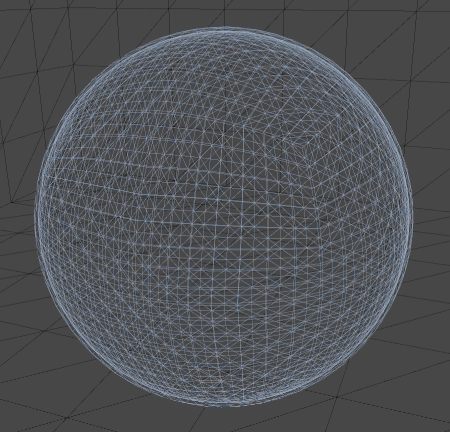It’s fairly high res as the more points you have with this method, the smoother the result.

[Sorry about the gifs being out of sync]

To be honest I could likely go higher res as I don’t need hundreds of these rolling around close to the camera. Even if we did, we can easily apply a LOD group to this.

Finally, I added a lower-res icosahedron for the outer bowl and some reflection probes.

This concludes the first part of the effect, we now have a a bowl we can alter the water level of, but there’s currently no water simulation, not is there any influence from the bowl’s movement. To do this, we’re going to look into some compute shaders.